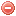# Thornthwaite equation

## Description

The Thornthwaite equation is a method developed by Thornthwaite (1948) based on an empirical approach in order to estimate potential evapotransipiration. Evapotranspiration is originally destinated to be computed on a monthly basis but daily estimations are possible as well (cf. Thornthwaite & Mather (1957).

## Formula

The potential evapotranspiration according to the Thornthwaite (1948) formulation $$PET_{Thorn}$$[mm/day] is calculated as follows (the equation for $$T>26$$ is an approximation of the original table proposed by Willmott et al. (1985) :

$PET_{Thorn}= \begin{cases} 0, & \mbox{if } T < 0\\ {16}\cdot\frac {N}{360}\cdot\bigg(\dfrac{10\cdot T}{I}\bigg)^a, & \mbox{if }0 \leqslant T \leqslant 26 \\ \frac {N}{360}\cdot\big(-415.85+30.5332.24 \cdot T - 0.43 \cdot T^2\big), & \mbox{if } T > 26\\ \end{cases}$

where $$N$$ is the duration of sunlight in hours, varying with season and latitude (cf. calculation of daylight hours after the FAO formulation, or Table 8 in Thornthwaite & Mather (1957), $$T$$ average daily air temperature [°C], and $$I$$a heat index calculated as follows:

$I=\displaystyle \sum_{Jan}^{Dec} \bigg(\dfrac{max[0,T_m]}{5}\bigg)^{1.514}$

where $$T_m$$ is monthly mean temperature [°C].

The exponent $$a$$ is calculated as follows:

$a=(6.75\cdot{10^{-7}}\cdot{I^3})-(7.71\cdot{10^{-5}}\cdot{I^2})+(0.01792\cdot{I})+(0.49239)$

An improved version of the daily Thornthwaite equation was proposed by Camargo et al. (1999) and Pereira & Pruitt (2004). Instead of average daily air temperature $$T$$ [°C], Camargo et al. (1999) suggested to use an "effective temperature" $$T_{ef}$$ [°C] given by:

$T_{ef}=\dfrac{1}{2}\cdot{k}\cdot(3\cdot{T_{max}}-T_{min})$

where $$k=0.72$$, $$T_{max}$$ is maximum daily temperature [°C], and $$T_{min}$$ minimum daily temperature [°C].

Pereira & Pruitt (2004) corrected this equation by including a day-night ratio:

$T_{ef}^*=T_{ef}\cdot\dfrac{N}{24-N}$

$\mbox{with }\dfrac{T_{max}+T_{min}}{2}\leqslant T_{ef}^* \leqslant T_{max}$

where $$T_{ef}^*$$ [°C] is the corrected $$T_{ef}$$, and $$N$$ the daylight hours.

## Reference

Thornthwaite (1948)
Thornthwaite & Mather (1957)
Willmott et al. (1985)
Camargo et al. (1999)
Pereira & Pruitt (2004)

# Penman equation

## Description

Penman (1948) developed an equation based on a more theoretical approach than Thornthwaite (1948) in order to estimate potential evaporation from open water. His equation has also been widely used to estimate evapotranspirations from vegetation covers. Shuttleworth (1993) reformulated the Penman equation in metric units.

## Formula

The potential evapotranspiration after Penman (1948) $$PET_{Pen}$$ [mm/day] is calculated as follows (Shuttleworth 1993):

$PET_{Pen}=\dfrac{\Delta}{\Delta+\gamma}\cdot\dfrac{R_n}{\lambda}+\dfrac{\gamma}{\Delta+\gamma}\cdot\dfrac{6.43\cdot(1+0.536\cdot{U})\cdot\Delta{e}}{\lambda}$

where $$\Delta$$ is the the slope of the saturation vapor pressure curve [kPa/Â°C], $$\gamma$$ the psychrometric constant [kPa/°C], $$R_n$$ the net radiation [MJ·m-2·d-1], $$\lambda$$ the latent heat of vaporization, $$U$$ the wind speed [m/s], and $$\Delta{e}$$ the vapor pressure deficit [kPa].

The slope of the saturation vapor pressure $$\Delta$$ is calculated as follows (Allen et al. 1998):

$\Delta=\dfrac{4098\cdot{0.6108\cdot{e^{\frac{17.27\cdot{T}}{T+237.3}}}}}{(T+237.3)^2}$

where $$T$$ is air temperature [°C].

The latent heat of vaporization $$\lambda$$ [MJ/kg] is calculated as follows (Shuttleworth 1993):

$\lambda=2.501-0.002361\cdot{T}$

The psychrometric constant $$\gamma$$ [kPa/°C] is calculated as follows (Shuttleworth 1993):

$\gamma=0.0016286\cdot\dfrac{p_{atm}}{\lambda}$

where $$p_{atm}$$ is the atmospheric pressure [kPa] and calculated as follows (Allen et al. 1998):

$p_{atm}=101.3\cdot\Big(\dfrac{293-0.0065\cdot{z}}{293}\Big)^{5.26}$

where $$z$$ is elevation above sea level [m].

Penman (1948)
Shuttleworth (1993)
Allen et al. (1998)

### Bibliography SearchNo results!

No results were found. Are you sure you searched for a tag?

### Symbols

 Variable Description Unit $$T$$ air temperature °C $$T_{dew}$$ dew point temperature °C $$H$$ air humidity % $$P$$ rainfall mm $$U$$ windspeed m/s $$w$$ days since last rain (or rain above threshold) d $$rr$$ days with consecutive rain d $$\Delta t$$ time increment d $$\Delta{e}$$ vapor pressure deficit kPa $$e_s$$ saturation vapor pressure kPa $$e_a$$ actual vapor pressure kPa $$p_{atm}$$ atmospheric pressure kPa $$PET$$ potential evapotranspiration mm/d $$r$$ soil water reserve mm $$r_s$$ surface water reserve mm $$EMC$$ equilibrium moisture content % $$DF$$ drought factor - $$N$$ daylight hours hr $$D$$ weighted 24-hr average moisture condition hr $$\omega$$ sunset hour angle rad $$\delta$$ solar declination rad $$\varphi$$ latitude rad $$Cc$$ cloud cover Okta $$J$$ day of the year (1..365/366) - $$I$$ heat index - $$R_n$$ net radiation MJ⋅m-2⋅d-1 $$R_a$$ daily extraterrestrial radiation MJ⋅m-2⋅d-1 $$R_s$$ solar radiation MJ⋅m-2⋅d-1 $$R_{so}$$ clear-sky solar radiation MJ⋅m-2⋅d-1 $$R_{ns}$$ net shortwave radiation MJ⋅m-2⋅d-1 $$R_{nl}$$ net longwave radiation MJ⋅m-2⋅d-1 $$\lambda$$ latent heat of vaporization MJ/kg $$z$$ elevation m a.s.l. $$d_r$$ inverse relative distance Earth-Sun - $$\alpha$$ albedo or canopy reflection coefficient - $$\Delta$$ slope of the saturation vapor pressure curve kPa/°C $$Cc$$ cloud cover eights $$ROS$$ rate of spread m/h $$RSF$$ rate of spread factor - $$WF$$ wind factor - $$WRF$$ water reserve factor - $$FH$$ false relative humidity - $$FAF$$ fuel availability factor - $$PC$$ phenological coefficient -

 Suffix Description $$-$$ mean / daily value $$_{max}$$ maximum value $$_{min}$$ minimum value $$_{12}$$ value at 12:00 $$_{13}$$ value at 13:00 $$_{15}$$ value at 15:00 $$_{m}$$ montly value $$_{y}$$ yearly value $$_{f/a}$$ value at fuel-atmosphere interface $$_{dur}$$ duration $$_{soil}$$ value at soil level

 Constant Description $$e$$ Euler's number $$\gamma$$ psychrometric constant $$G_{SC}$$ solar constant $$\sigma$$ Stefan-Bolzmann constant